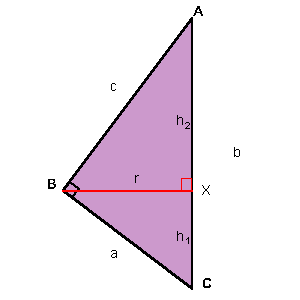You may also likePolycircles

Show that for any triangle it is always possible to construct 3 touching circles with centres at the vertices. Is it possible to construct touching circles centred at the vertices of any polygon?Nim

Start with any number of counters in any number of piles. 2 players take it in turns to remove any number of counters from a single pile. The loser is the player who takes the last counter.Loopy

Investigate sequences given by $a_n = \frac{1+a_{n-1}}{a_{n-2}}$ for different choices of the first two terms. Make a conjecture about the behaviour of these sequences. Can you prove your conjecture?

In a Spin

Age 14 to 16 Challenge Level:

Correct solutions were received from Joshua T. and Andrew I. Congratulations to both of you. I have used a mixture of the solutions to form the basis of what follows.

The first thing to observe is that by rotating the triangle about the hypotenuse I obtain two cones having the same base and opposite vertices.To find the volume of a cone you need its height and the radius of its base. So you need to calculate the radius and the height of each cone.

$$V = \frac{1}{3}\pi r^2 h$$

The radius of the base of the cones is the altitude of the right-angled triangle ($r$), and the corresponding heights are $AX$ and $CX$.

Working directly on the general case - as it seems simpler. Let the sides of the triangle be $a$, $c$ and $b$ ($b$- the hypotenuse).

\begin{eqnarray}V &=& \frac{1}{3}\pi r^2 h \\ &=& \frac{1}{3}\pi r^2 h_{1}+\frac{1}{3}\pi r^2 h_{2}\\ &=&\frac{1}{3}\pi r^2 (h_{1}+h_{2}) \\ &=& \frac{1}{3}\pi r^2 b \end{eqnarray}

Finding the area of a right-angled triangle in two ways:

$$\frac{ac}{2}=\frac{rb}{2}$$

Therefore $$r = \frac{ac}{b}$$

Substituting for $r$ in the equation for the volume you get:

\begin{eqnarray} V &=& \frac{1}{3}\pi r^2 b \\ &=& \frac{1}{3}\pi \left( {\frac{{ac}}{b}} \right)^2 b \\ &=& \frac{1}{3}\pi \frac{{(ac)}^2}{b}\end{eqnarray}

From here you can substitute for the particular case given at the start of the problem.